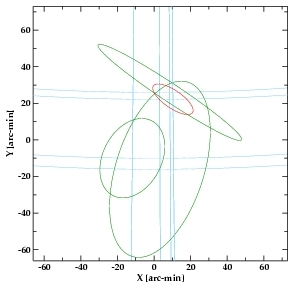## Source Position Errors in the Master Sources Table

### Summary

The position and 2-dimensional positional uncertainty of a source listed in the Master Sources Table represents the best estimate of the source position based on several independent measures, where the master sources table entry is the merged result of multiple stacked observation detections of the same source. To determine the best estimate of the position of a source from previous independent estimates of its position, we employ a 2-dimensional optimal weighting formalism to statistically average the detection positions resulting from the set of individual stacked observations of the source. We decided to use this technique because it offers an improved estimate of source position where simple averaging fails, e.g., where the area defining the detection position varies significantly from measure to measure. We express the uncertainties of the estimates in the form of error ellipses centered upon the estimated detection positions.

### Input Ellipses and the Combined Ellipse### Input Ellipses and the Combined Ellipse

An example of input ellipses (green) and the combined ellipse (red). The x and y values represent tangent plane coordinates.

The Stacked Observation Detections Table error ellipses that are combined to produce the best estimate error ellipse for the Master Sources Table entry are determined from the best-fitting ellipse to the position-uncertainty–fit-statistic surface computed from the MLE's Markov chain Monte Carlo (MCMC) draws, as described in the page "Source Position Errors in the Stacked Observation Detections Table".

The use of the multivariate optimal weighting formalism used in the CSC, described below, is discussed in John Davis' memo "Combining Error Ellipses".

### Error Ellipses

#### Multivariate Optimal Weighting

The multivariate optimal weighting formalism used to combine error ellipses can be distilled to the following formula

where $$X_{a}$$ represents the $$a\mathrm{th}$$ estimate of the mean of the 2-dimensional source position, $$\sigma_{a}$$ denotes the $$2 \times 2$$ covariance matrix associated with this estimate, and

#### Tangent Plane Projection

Before the covariance matrix, $$\sigma$$, may be computed—permitting the ellipses to be combined via the multivariate weighted sum—the error ellipses must be mapped from the celestial sphere onto a common tangent plane. The $$a\mathrm{th}$$ estimate of the source position is specified as a confidence-ellipse centered upon the celestial coordinate, $$(\alpha_{a},\delta_{a})$$, with the major axis making an angle $$\theta$$ $$(-\pi \leq; \theta < \pi)$$ with respect to the local line of declination at the center of the ellipse. The tangent plane coordinates $$(x_{a},y_{a})$$ of the center of the $$a\mathrm{th}$$ ellipse are

where $$\hat{p}_{a}$$ is a unit-vector on the celestial sphere corresponding to the $$a\mathrm{th}$$ estimate of the celestial coordinate $$(\alpha_{a},\delta_{a})$$ defining the source position, given by

Similar equations give the end-point positions $$\hat{p}_{a}^{minor}$$ and $$\hat{p}_{a}^{major}$$ of the semi-minor and semi-major axes of each ellipse:

and

where $$\phi_{a}^{minor}$$ is the arc-length of the semi-minor axis and $$\phi_{a}^{major}$$ is that of the semi-major axis.

$$\hat{p}_{0}$$ denotes the position on the celestial sphere where a tangent plane is to be erected; $$\hat{p}_{0}$$ is taken to be the arithmetic mean of the ellipse centers $$\hat{p}_{a}$$, i.e.,

A coordinate system may be given to the tangent plane with the origin at $$\hat{p}_{0}$$ and orthonormal basis vectors $$\hat{e}_{x}$$ and $$\hat{e}_{y}$$ parallel to the local lines of right ascension and declination at $$\hat{p}_{0}$$, i.e.,

and

where $$(\alpha_{0},\delta_{0})$$ are the celestial coordinates that correspond to $$\hat{p}_{0}$$.

The tangent plane coordinates that correspond to the end-point positions $$\hat{p}_{a}^{major}$$ and $$\hat{p}_{a}^{minor}$$ of the semi-major and semi-minor axes of the ellipse are denoted by $$(x_{a}^{major},y_{a}^{major})$$ and $$(x_{a}^{minor},y_{a}^{minor})$$. The lengths of the semi-major and semi-minor axes in the tangent plane are given by

and

respectively. Finally, the angle that the semi-major axis makes with respect to the local line of declination is

Armed with these relations, it is easy to compute the tangent plane projections of the error ellipses.

### Computing Covariance Matrices

Three of the parameters specifying the geometry of each projected error ellipse are the semi-major and semi-minor axis lengths, and the position angle $$\theta$$ that the major axis of the ellipse makes with respect to the tangent plane y-axis. The semi-major and semi-minor axis lengths correspond to the $$1\sigma$$ confidence intervals along these axes. More specifically, in a basis whose origin is at the center of the ellipse, and whose y-axis is along the major axis of the ellipse, the correlation matrix is

Here, $$\sigma^{\prime}_{1}$$ is the $$1\sigma$$ confidence value along the minor axis of the ellipse, and $$\sigma^{\prime}_{2}$$ is that along the major axis $$(\sigma^{\prime}_{2} \geq \sigma^{\prime}_{1})$$. The form of the covariance matrix $$\sigma$$ in the unrotated system follows from

where $$\mathcal{R}$$ is a rotation matrix that transforms a vector $$X$$ to $$X^{\prime} = \mathcal{R}X$$. Here, $$\mathcal{R}$$ is defined as

which yields

At this point, the lengths of the semi-major and semi-minor axes of the source position error ellipses in the tangent plane, $$\sigma^{\prime}_{1}$$ and $$\sigma^{\prime}_{2}$$ defined at the end of the previous section, may be input to the covariance matrix, $$\sigma$$ above, permitting the error ellipses to be combined via the multivariate weighted sum:

This process produces the geometric parameters of a combined 2-D error ellipse on the tangent plane $$(X)$$, which are recorded in the "Source Position and Position Errors" fields err_ellipse_r0, err_ellipse_r1, and err_ellipse_ang in the Master Chandra Sources Table.